# Mass Spring Damper Example¶The equation of motion is given as:

$m \ddot{x}(t) + c \dot{x}(t) + k x(t) = u(t)$

You are given the following physical parameters.

• c=2 N/(m/s)
• k=2 N/m
• m=1 kg

## Laplace Method for Matrix Exponential¶

In :
import matplotlib.pyplot as plt
%matplotlib inline
import sympy

sympy.init_printing()
t = sympy.symbols('t', real=True)
eps = 1e-10  # where to start plotting, since we assume positive t
s = sympy.symbols('s')
c = 1
k = 1

A = sympy.Matrix([[0, 1], [-k, -c]])
B = sympy.Matrix([, ])
C = sympy.Matrix([[1, 0]])
D = sympy.Matrix([])
(A, B, C, D)

Out:
$\displaystyle \left( \left[\begin{matrix}0 & 1\\-1 & -1\end{matrix}\right], \ \left[\begin{matrix}0\\1\end{matrix}\right], \ \left[\begin{matrix}1 & 0\end{matrix}\right], \ \left[\begin{matrix}0\end{matrix}\right]\right)$

$G(s) = C(sI - A)^{-1}B + D$

In :
N = (s*sympy.eye(2) - A).inv()
G = (C*N*B + D).expand()
G.simplify()
G = G
G

Out:
$\displaystyle \frac{1}{s^{2} + s + 1}$

Remember from the Laplace table:

$\mathcal{L}^{-1}\left[ \dfrac{1}{s - a} \right] = e^{at}$

The same is true for the matrix version of this Laplace transform:

$\mathcal{L}^{-1}\left[ (sI- A)^{-1} \right] = e^{At}$

In :
eAt = sympy.inverse_laplace_transform((s*sympy.eye(2) - A).inv(), s, t)
eAt

Out:
$\displaystyle \left[\begin{matrix}\frac{\sqrt{3} \left(\sin{\left(\frac{\sqrt{3} t}{2} \right)} + \sqrt{3} \cos{\left(\frac{\sqrt{3} t}{2} \right)}\right) e^{- \frac{t}{2}} \theta\left(t\right)}{3} & \frac{2 \sqrt{3} e^{- \frac{t}{2}} \sin{\left(\frac{\sqrt{3} t}{2} \right)} \theta\left(t\right)}{3}\\- \frac{2 \sqrt{3} e^{- \frac{t}{2}} \sin{\left(\frac{\sqrt{3} t}{2} \right)} \theta\left(t\right)}{3} & \frac{\sqrt{3} \left(- \sin{\left(\frac{\sqrt{3} t}{2} \right)} + \sqrt{3} \cos{\left(\frac{\sqrt{3} t}{2} \right)}\right) e^{- \frac{t}{2}} \theta\left(t\right)}{3}\end{matrix}\right]$
In :
sympy.plot(sympy.Heaviside(t), (t, -1, 1))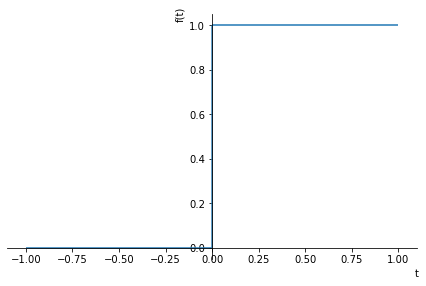Out:
<sympy.plotting.plot.Plot at 0x7f74c3dd4d00>

## Spectral Method for Matrix Exponential¶

The approach here will be to use the diagonalization of A.

In :
A

Out:
$\displaystyle \left[\begin{matrix}0 & 1\\-1 & -1\end{matrix}\right]$
In :
evects = sympy.Matrix.eigenvects(A)
evects

Out:
$\displaystyle \left[ \left( - \frac{1}{2} - \frac{\sqrt{3} i}{2}, \ 1, \ \left[ \left[\begin{matrix}- \frac{2}{1 + \sqrt{3} i}\\1\end{matrix}\right]\right]\right), \ \left( - \frac{1}{2} + \frac{\sqrt{3} i}{2}, \ 1, \ \left[ \left[\begin{matrix}- \frac{2}{1 - \sqrt{3} i}\\1\end{matrix}\right]\right]\right)\right]$

$T = \left[ \xi_1 \xi_2 \right]$

Diagonolization:

$A = T diag(\lambda_1, \lambda_2) T^{-1}$

In :
T = sympy.Matrix.hstack(evects, evects)
T

Out:
$\displaystyle \left[\begin{matrix}- \frac{2}{1 + \sqrt{3} i} & - \frac{2}{1 - \sqrt{3} i}\\1 & 1\end{matrix}\right]$
In :
T_inv = T.inv()
T_inv.simplify()
T_inv

Out:
$\displaystyle \left[\begin{matrix}\frac{\frac{\sqrt{3}}{3} - i}{\sqrt{3} + i} & \frac{1}{2} - \frac{\sqrt{3} i}{6}\\\frac{\sqrt{3} i}{3} & \frac{1}{2} + \frac{\sqrt{3} i}{6}\end{matrix}\right]$
In :
Lambda = sympy.diag(evects, evects)
Lambda

Out:
$\displaystyle \left[\begin{matrix}- \frac{1}{2} - \frac{\sqrt{3} i}{2} & 0\\0 & - \frac{1}{2} + \frac{\sqrt{3} i}{2}\end{matrix}\right]$

Let's check that our diagonalization of A worked as expected.

In :
A_check = (T*Lambda*T.inv())
sympy.expand(A_check, complex=True), A

Out:
$\displaystyle \left( \left[\begin{matrix}0 & 1\\-1 & -1\end{matrix}\right], \ \left[\begin{matrix}0 & 1\\-1 & -1\end{matrix}\right]\right)$

We can calculate the exponential of the diagonal matrix containing the eigen values, it is just the exponential of each element of the diagonal.

In :
LambdaExp = sympy.diag(sympy.exp(evects*t), sympy.exp(evects*t))
LambdaExp

Out:
$\displaystyle \left[\begin{matrix}e^{t \left(- \frac{1}{2} - \frac{\sqrt{3} i}{2}\right)} & 0\\0 & e^{t \left(- \frac{1}{2} + \frac{\sqrt{3} i}{2}\right)}\end{matrix}\right]$
In :
eAt_spec = (T*LambdaExp*T_inv).expand(complex=True)*sympy.Heaviside(t)
eAt_spec

Out:
$\displaystyle \left[\begin{matrix}\left(\frac{\sqrt{3} e^{- \frac{t}{2}} \sin{\left(\frac{\sqrt{3} t}{2} \right)}}{3} + e^{- \frac{t}{2}} \cos{\left(\frac{\sqrt{3} t}{2} \right)}\right) \theta\left(t\right) & \frac{2 \sqrt{3} e^{- \frac{t}{2}} \sin{\left(\frac{\sqrt{3} t}{2} \right)} \theta\left(t\right)}{3}\\- \frac{2 \sqrt{3} e^{- \frac{t}{2}} \sin{\left(\frac{\sqrt{3} t}{2} \right)} \theta\left(t\right)}{3} & \left(- \frac{\sqrt{3} e^{- \frac{t}{2}} \sin{\left(\frac{\sqrt{3} t}{2} \right)}}{3} + e^{- \frac{t}{2}} \cos{\left(\frac{\sqrt{3} t}{2} \right)}\right) \theta\left(t\right)\end{matrix}\right]$
In :
eAt

Out:
$\displaystyle \left[\begin{matrix}\frac{\sqrt{3} \left(\sin{\left(\frac{\sqrt{3} t}{2} \right)} + \sqrt{3} \cos{\left(\frac{\sqrt{3} t}{2} \right)}\right) e^{- \frac{t}{2}} \theta\left(t\right)}{3} & \frac{2 \sqrt{3} e^{- \frac{t}{2}} \sin{\left(\frac{\sqrt{3} t}{2} \right)} \theta\left(t\right)}{3}\\- \frac{2 \sqrt{3} e^{- \frac{t}{2}} \sin{\left(\frac{\sqrt{3} t}{2} \right)} \theta\left(t\right)}{3} & \frac{\sqrt{3} \left(- \sin{\left(\frac{\sqrt{3} t}{2} \right)} + \sqrt{3} \cos{\left(\frac{\sqrt{3} t}{2} \right)}\right) e^{- \frac{t}{2}} \theta\left(t\right)}{3}\end{matrix}\right]$

## Response given initial conditions¶

$\vec{x} = \begin{bmatrix}x \\ \dot{x} \end{bmatrix}$

$\vec{x}(t) = e^{At}\vec{x}(0) + \int_0^t e^{A(t - \tau)} B u(\tau) d\tau$

$\vec{y}(t) = C \vec{x}(t) + D\vec{u}(t)$

In :
x0 = sympy.Matrix([1, 0])
x0

Out:
$\displaystyle \left[\begin{matrix}1\\0\end{matrix}\right]$
In :
x = eAt*x0
x

Out:
$\displaystyle \left[\begin{matrix}\frac{\sqrt{3} \left(\sin{\left(\frac{\sqrt{3} t}{2} \right)} + \sqrt{3} \cos{\left(\frac{\sqrt{3} t}{2} \right)}\right) e^{- \frac{t}{2}} \theta\left(t\right)}{3}\\- \frac{2 \sqrt{3} e^{- \frac{t}{2}} \sin{\left(\frac{\sqrt{3} t}{2} \right)} \theta\left(t\right)}{3}\end{matrix}\right]$
In :
sympy.plot(x, (t, eps, 10))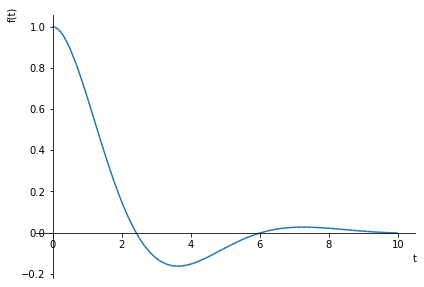Out:
<sympy.plotting.plot.Plot at 0x7f753055aac0>
In :
sympy.plot(x)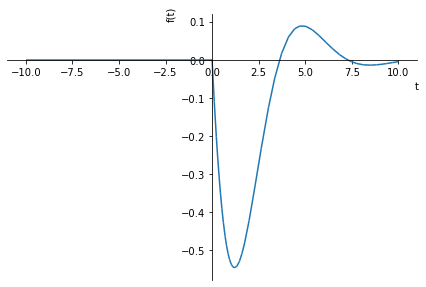Out:
<sympy.plotting.plot.Plot at 0x7f7530cd0550>

# Final Value Theorem¶

$G(s) = Y(s)/U(s)$

$\lim\limits_{t \rightarrow \infty} x(t) = \lim\limits_{s \rightarrow 0} s X(s)$

In :
G

Out:
$\displaystyle \frac{1}{s^{2} + s + 1}$

## Unit step of magnitude 3¶

$X = G\cdot U$

$X = (X/U)\cdot U$

In :
U1 = 3/s
X1 = G*U1
X1

Out:
$\displaystyle \frac{3}{s \left(s^{2} + s + 1\right)}$
In :
u1 = sympy.inverse_laplace_transform(U1, s, t)
u1

Out:
$\displaystyle 3 \theta\left(t\right)$
In :
x1_final = sympy.simplify(s*X1).subs(s, 0)
x1_final

Out:
$\displaystyle 3$
In :
x1 = sympy.inverse_laplace_transform(sympy.apart(X1, full=True), s, t)
x1

Out:
$\displaystyle 3 \theta\left(t\right) - 2 \sqrt{3} e^{- \frac{t}{2}} \sin{\left(\frac{\sqrt{3} t}{2} + \frac{\pi}{3} \right)} \theta\left(t\right)$
In :
sympy.plot(x1, x1_final, (t, eps, 10))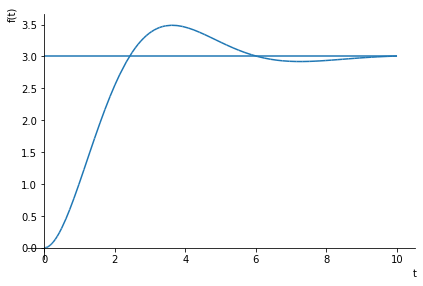Out:
<sympy.plotting.plot.Plot at 0x7f7530ff7610>

## Impulse of magnitude 2¶

In :
U2 = 2
X2 = G*U2
X2

Out:
$\displaystyle \frac{2}{s^{2} + s + 1}$
In :
u2 = sympy.inverse_laplace_transform(U2, s, t)
u2

Out:
$\displaystyle 2 \delta\left(t\right)$
In :
x2_final = (s*X2).subs(s, 0)
x2_final

Out:
$\displaystyle 0$
In :
s*X2

Out:
$\displaystyle \frac{2 s}{s^{2} + s + 1}$
In :
x2 = sympy.inverse_laplace_transform(X2, s, t).expand(complex=True)
x2

Out:
$\displaystyle \frac{4 \sqrt{3} e^{- \frac{t}{2}} \sin{\left(\frac{\sqrt{3} t}{2} \right)} \theta\left(t\right)}{3}$
In :
sympy.plot(x2, x2_final, (t, eps, 10))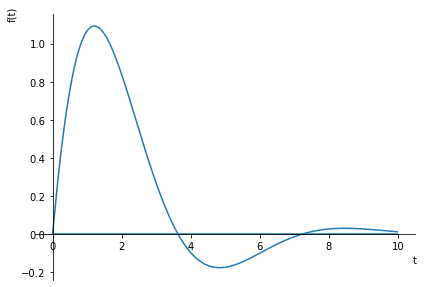Out:
<sympy.plotting.plot.Plot at 0x7f75310aafa0>